Updating search results...

# 24 Results

View
Selected filters:
• Trigonometry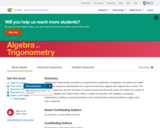Unrestricted Use
CC BY
Rating
0.0 stars

Algebra and Trigonometry provides a comprehensive exploration of algebraic principles and meets scope and sequence requirements for a typical introductory algebra and trigonometry course. The modular approach and the richness of content ensures that the book meets the needs of a variety of courses. Algebra and Trigonometry offers a wealth of examples with detailed, conceptual explanations, building a strong foundation in the material before asking students to apply what they’ve learned.

Subject:
Algebra
Mathematics
Trigonometry
Material Type:
Textbook
Provider:
Rice University
Provider Set:
OpenStax College
Author:
David Lippman
Jay Abramson
Jean-Marie Magnier
Melonie Rasmussen
Nicholas Belloit
Rachael Gross
Rick Norwood
Valeree Falduto
01/29/2015Conditional Remix & Share Permitted
CC BY-NC-SA
Rating
0.0 stars

This task was developed by high school and postsecondary mathematics and design/pre-construction educators, and validated by content experts in the Common Core State Standards in mathematics and the National Career Clusters Knowledge & Skills Statements. It was developed with the purpose of demonstrating how the Common Core and CTE Knowledge & Skills Statements can be integrated into classroom learning - and to provide classroom teachers with a truly authentic task for either mathematics or CTE courses.

Subject:
Applied Science
Architecture and Design
Geometry
Mathematics
Trigonometry
Material Type:
Activity/Lab
Assessment
Homework/Assignment
Lesson Plan
Provider:
National Association of State Directors of Career Technical Education Consortium
Provider Set:
Career Technical Education
10/26/2021Unrestricted Use
CC BY
Rating
0.0 stars

College Algebra provides a comprehensive exploration of algebraic principles and meets scope and sequence requirements for a typical introductory algebra course. The modular approach and richness of content ensure that the book meets the needs of a variety of courses. College Algebra offers a wealth of examples with detailed, conceptual explanations, building a strong foundation in the material before asking students to apply what theyve learned.

Subject:
Algebra
Mathematics
Trigonometry
Material Type:
Textbook
Provider:
Rice University
Provider Set:
OpenStax College
Author:
David Lippman
Jay Abramson
Jean-Marie Magnier
Melonie Rasmussen
Nicholas Belloit
Rachael Gross
Rick Norwood
Valeree Falduto
01/29/2015Conditional Remix & Share Permitted
CC BY-NC-SA
Rating
0.0 stars

This College Algebra text will cover a combination of classical algebra and analytic geometry, with an introduction to the transcendental exponential and logarithmic functions. If mathematics is the language of science, then algebra is the grammar of that language. Like grammar, algebra provides a structure to mathematical notation, in addition to its uses in problem solving and its ability to change the appearance of an expression without changing the value.

Subject:
Algebra
Mathematics
Trigonometry
Material Type:
Textbook
Author:
Richard W. Beveridge
12/05/2019Conditional Remix & Share Permitted
CC BY-NC-SA
Rating
0.0 stars

This book was designed as an introductory trigonometry textbook for college students with the explicit goal of reducing textbook costs.

Subject:
Algebra
Mathematics
Trigonometry
Material Type:
Textbook
Provider:
Stitz-Zeager Open Source Mathematics
Author:
Carl Stitz
Jeff Zeager
07/15/2011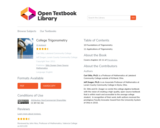Conditional Remix & Share Permitted
CC BY-NC-SA
Rating
0.0 stars

This book was designed as an introductory trigonometry textbook for college students with the explicit goal of reducing textbook costs.

Subject:
Algebra
Mathematics
Trigonometry
Material Type:
Textbook
Author:
Carl Stitz
Jeff Zeager
07/15/2011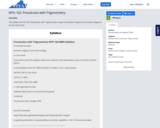Unrestricted Use
CC BY
Rating
0.0 stars

The syllabus for&nbsp;MTH 162: Precalculus with Trigonometry, taught at Northern Virginia Community College by Dr.&nbsp;Don Goral, PhD.

Subject:
Calculus
Mathematics
Trigonometry
Material Type:
Syllabus
Author:
Virtual Library of Virginia (VIVA)
08/20/2021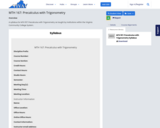Unrestricted Use
CC BY
Rating
0.0 stars

A syllabus for MTH 167: Precalculus with Trigonometry as taught by institutions within the Virginia Community College System.

Subject:
Calculus
Mathematics
Trigonometry
Material Type:
Syllabus
Author:
Virtual Library of Virginia (VIVA)
05/18/2021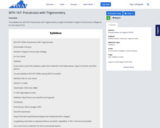Unrestricted Use
CC BY
Rating
0.0 stars

The syllabus for&nbsp;MTH 167: Precalculus with Trigonometry, taught at Northern Virginia Community College&nbsp;by Dr.&nbsp;Don Goral, Ph.D.

Subject:
Calculus
Mathematics
Trigonometry
Material Type:
Syllabus
Author:
Virtual Library of Virginia (VIVA)
08/20/2021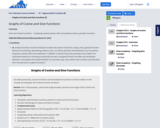Conditional Remix & Share Permitted
CC BY-NC
Rating
0.0 stars

Sine and Cosine Functions - amplitude, period, phase-shift, sinusoidal functions, periodic functionsTMM 002 PRECALCULUS (Revised March 21, 2017)1. Functions: 1a. Analyze functions. Routine analysis includes discussion of domain, range, zeros, general function behavior (increasing, decreasing, extrema, etc.), as well as periodic characteristics such as period, frequency, phase shift, and amplitude. In addition to performing rote processes, the student can articulate reasons for choosing a particular process, recognize function families and anticipate behavior, and explain the implementation of a process (e.g., why certain real numbers are excluded from the domain of a given function).*

Subject:
Calculus
Mathematics
Trigonometry
Material Type:
Module
Author:
Ohio Open Ed Collaborative
11/02/2020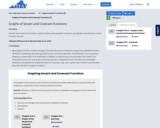Conditional Remix & Share Permitted
CC BY-NC
Rating
0.0 stars

Secant and Cosecant Functions - period, phase-shift, periodic functions, asymptotes, sine function, cosine function, domainTMM 002 PRECALCULUS (Revised March 21, 2017)1. Functions: 1a. Analyze functions. Routine analysis includes discussion of domain, range, zeros, general function behavior (increasing, decreasing, extrema, etc.), as well as periodic characteristics such as period, frequency, phase shift, and amplitude. In addition to performing rote processes, the student can articulate reasons for choosing a particular process, recognize function families and anticipate behavior, and explain the implementation of a process (e.g., why certain real numbers are excluded from the domain of a given function).*

Subject:
Calculus
Mathematics
Trigonometry
Material Type:
Module
Author:
Ohio Open Ed Collaborative
11/02/2020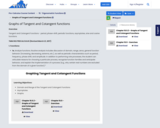Conditional Remix & Share Permitted
CC BY-NC
Rating
0.0 stars

Tangent and Cotangent Functions - period, phase-shift, periodic functions, asymptotes, sine and cosine functionsTMM 002 PRECALCULUS (Revised March 21, 2017)1. Functions: 1a. Analyze functions. Routine analysis includes discussion of domain, range, zeros, general function behavior (increasing, decreasing, extrema, etc.), as well as periodic characteristics such as period, frequency, phase shift, and amplitude. In addition to performing rote processes, the student can articulate reasons for choosing a particular process, recognize function families and anticipate behavior, and explain the implementation of a process (e.g., why certain real numbers are excluded from the domain of a given function).*

Subject:
Calculus
Higher Education
Mathematics
Trigonometry
Material Type:
Module
Author:
Ohio Open Ed Collaborative
11/02/2020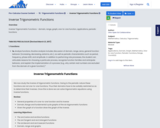Conditional Remix & Share Permitted
CC BY-NC
Rating
0.0 stars

Inverse Trigonometric Functions - domain, range, graph, one-to-one function, applications, periodic functions&nbsp;TMM 002 PRECALCULUS (Revised March 21, 2017)1. Functions: 1a. Analyze functions. Routine analysis includes discussion of domain, range, zeros, general function behavior (increasing, decreasing, extrema, etc.), as well as periodic characteristics such as period, frequency, phase shift, and amplitude. In addition to performing rote processes, the student can articulate reasons for choosing a particular process, recognize function families and anticipate behavior, and explain the implementation of a process (e.g., why certain real numbers are excluded from the domain of a given function).*

Subject:
Calculus
Mathematics
Trigonometry
Material Type:
Module
Author:
Ohio Open Ed Collaborative
11/02/2020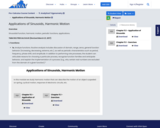Conditional Remix & Share Permitted
CC BY-NC
Rating
0.0 stars

Sinusoidal function, harmonic motion, periodic functions, applications.TMM 002 PRECALCULUS (Revised March 21, 2017)1. Functions: 1a. Analyze functions. Routine analysis includes discussion of domain, range, zeros, general function behavior (increasing, decreasing, extrema, etc.), as well as periodic characteristics such as period, frequency, phase shift, and amplitude. In addition to performing rote processes, the student can articulate reasons for choosing a particular process, recognize function families and anticipate behavior, and explain the implementation of a process (e.g., why certain real numbers are excluded from the domain of a given function).*

Subject:
Calculus
Mathematics
Physics
Trigonometry
Material Type:
Module
Author:
Ohio Open Ed Collaborative
11/02/2020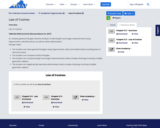Conditional Remix & Share Permitted
CC BY-NC
Rating
0.0 stars

Law of CosinesTMM 002 PRECALCULUS (Revised March 21, 2017)2c. Analyze general triangles. Routine analysis of side lengths and angle measurements using trigonometric ratios/functions, as well as other relationships.*Sample Tasks:The student can solve general triangles using trigonometric ratios and relationships including laws of sine and cosine.The student can compare similar triangles.The student can compute length and angle measurements inside complex drawings involving multiple geometric objects.The student can algebraically describe relationships inside complex drawings involving multiple geometric objects.

Subject:
Calculus
Geometry
Mathematics
Trigonometry
Material Type:
Module
Author:
Ohio Open Ed Collaborative
11/02/2020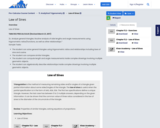Conditional Remix & Share Permitted
CC BY-NC
Rating
0.0 stars

Law of SinesTMM 002 PRECALCULUS (Revised March 21, 2017)2c. Analyze general triangles. Routine analysis of side lengths and angle measurements using trigonometric ratios/functions, as well as other relationships.*Sample Tasks:The student can solve general triangles using trigonometric ratios and relationships including laws of sine and cosine.The student can compare similar triangles.The student can compute length and angle measurements inside complex drawings involving multiple geometric objects.The student can algebraically describe relationships inside complex drawings involving multiple geometric objects.

Subject:
Calculus
Geometry
Higher Education
Mathematics
Trigonometry
Material Type:
Module
Author:
Ohio Open Ed Collaborative
11/02/2020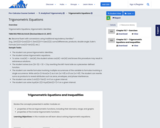Conditional Remix & Share Permitted
CC BY-NC
Rating
0.0 stars

Trigonometric Equations, trigonometric identitiesTMM 002 PRECALCULUS (Revised March 21, 2017)4c. Become fluent with conversions using traditional equivalency families.*(e.g., (sin(𝑡))2+(cos(𝑡))2=1; (tan(𝑡))2+1=(sec(𝑡))2; sums/differences; products; double angle; Euler&rsquo;s Formula (𝑒𝑖𝜃=cos(𝜃)+𝑖sin(𝜃)); etc.)Sample Tasks:The student can prove trigonometric identities.The student solves trigonometric equations.To solve &radic;cos(4𝑡) = &radic;sin(4𝑡), the student solves cos(4𝑡) =sin(4𝑡) and knows this procedure may result in extraneous solutions.The student solves |cos (2𝜃&minus;3)| + 32 = 2 by rewriting the left-hand side as a piecewise-defined function.The student can rewrite formulas involving multiple occurrences of the variable to formulas involving a single occurrence. Write 𝑎sin(𝑤 𝑡)+𝑏cos(𝑤 𝑡) as 𝐴 sin (𝑤 𝑡+𝐵) or 𝐵 cos (𝑤 𝑡+𝐵). The student can rewrite sums as products to reveal attributes such as zeros, envelopes, and phase interference.The student can solve 2 𝑠𝑖𝑛2(𝑡)+7sin(𝑡)&minus;4=0 on a given interval.The student can solve 𝑙𝑜𝑔4(sin (𝑡))+𝑙𝑜𝑔4(2sin(𝑡)+7)=1 on a given interval.&nbsp;

Subject:
Calculus
Mathematics
Trigonometry
Material Type:
Module
Author:
Ohio Open Ed Collaborative
11/02/2020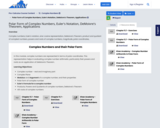Conditional Remix & Share Permitted
CC BY-NC
Rating
0.0 stars

Complex numbers, Euler&#39;s notation, sine-cosine representation, DeMoivre&#39;s Theorem, product and quotient of complext numbers, powers and roots of complex numbers, magnitude, polar coordinates,

Subject:
Calculus
Mathematics
Trigonometry
Material Type:
Module
Author:
Ohio Open Ed Collaborative
11/02/2020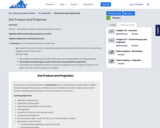Conditional Remix & Share Permitted
CC BY-NC
Rating
0.0 stars

Vectors - dot product, projection, decomposition of a vectorTMM 002 PRECALCULUS (Revised March 21, 2017)AdditionalOptional Learning Outcomes:2. Geometry: The successful Precalculus student can:2e. Interpret the result of vector computations geometrically and within the confines of a particular applied context (e.g., forces).Sample Tasks:The student can define vectors, their arithmetic, their representation, and interpretations.The student can decompose vectors into normal and parallel components.The student can interpret the result of a vector computation as a change in location in the plane or as the net force acting on an object.

Subject:
Engineering
Geometry
Higher Education
Mathematics
Trigonometry
Material Type:
Module
Author:
Ohio Open Ed Collaborative
11/02/2020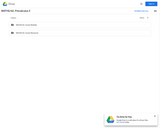Unrestricted Use
CC BY
Rating
0.0 stars

This course will cover families of trigonometric functions, their inverses, properties, graphs, and applications. Additionally we will study trigonometric equations and identities, the laws of sines and cosines, polar coordinates and graphs, parametric equations and elementary vector operations.Login: guest_oclPassword: ocl

Subject:
Mathematics
Trigonometry
Material Type:
Full Course
Homework/Assignment
Lecture Notes
Lesson Plan